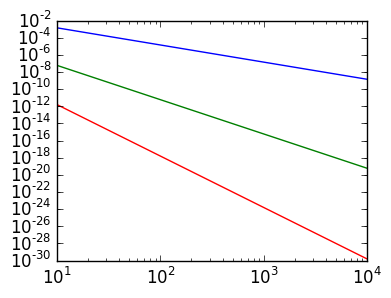# Lab 10: Romberg integration¶

In lectures we saw that the error in the Trapezium rule satisfies

$$\int_0^1 f(x) dx - Q_n[f] = -{1 \over 12 n^2}({f'(1) - f'(0)}) + O(n^{-4})$$

where

$$Q_n[f] \triangleq {1 \over n}\left[{f(0) \over 2} + \sum_{j=1}^{n-1} f(x_j) + {f(1) \over 2}\right]$$

and, as always, $x_k = k h$ for $h=1/n$.

This lecture explores ways we can use this formula to accelerate convergence when we don't know the derivatives of $f$.

Warning These are still not the best numerical integration schemes when one can choose the grid! But they are fairly powerful when we are restricted only knowing the data at evenly spaced points.

It may be convenient to use the trap routine from lectures:

In :
function trap(f,a,b,n)
h=(b-a)/n
x=linspace(a,b,n+1)

v=f(x)
h/2*v+sum(v[2:end-1])*h+h/2*v[end]
end;


## Richardson Extrapolation¶

The idea behind Romberg quadrature is to use Richardson Extrapolation, which is a general approach for convergence accelleration. Richardson extrapolation is based on the observation that the constant in the first term of the does not depend on $n$. That is, we have the error

$$\int_0^1 f(x) dx - Q_n[f] = {\alpha \over n^2} + O(n^{-4})$$

where $\alpha= -{f'(1) - f'(0) \over 12}$ only depends on $f$. This means that, even if we don't know $\alpha$, we can cancel it out by evaluating the Trapezodial rule for different choices of $n$.

Exercise 1(a) What is the first term of the error for $Q_{2n}[f]$? Show that subtracting out this term does give $O(n^{-4})$, for $f(x) = e^x$.

In :
ex=quadgk(exp,0,1)

n=10;
ex-trap(exp,0,1,2*n) -(-1/(12*4*n^2)*(exp(1)-exp(0)))

Out:
1.491475287951306e-8

Exercise 1(b) Choose constants $A$ and $B$ independent of $n$ so that

$$AQ_n[f] +B Q_{2n}[f] = \int_0^1 f(x) dx + O(n^{-4})$$

(Hint: we clearly have $A+B =1$. What other condition is there? Reduce this to a 2 x 2 linear system.)

Exercise 1(c) Demonstrate that this does indeed work numerically.

In :
n=20;

ex-(-trap(exp,0,1,n)/3+(1+1/3)*trap(exp,0,1,2n))

Out:
-3.728632513855246e-9

Exercise 2(a) What is the first and second term of the error for $Q_{n}[f]$, $Q_{2n}[f]$ $Q_{3n}[f]$?

Exercise 2(b) Advanced Set up a linear system to choose constants $A$, $B$, and $C$ independent of $n$ so that we have

$$A Q_n[f] + BQ_{2n}[f] + CQ_{3n}[f] = \int_0^1 f(x) dx + O(n^{-6})$$

Demonstrate this holds true numerically.

In :
A,B,C=[1 1 1;
1 1/4 1/9;
1 1/2^4 1/3^4]\[1;0;0]

Out:
3-element Array{Float64,1}:
0.0416667
-1.06667
2.025    
In :
n=20
ex-(A*trap(exp,0,1,n)+B*trap(exp,0,1,2n)+C*trap(exp,0,1,3n))

Out:
-2.5757174171303632e-14

The error quickly reaches close to machine epsilon, and so it's difficult to see the faster convegence. We can overcome this limitation using BigFloat.

Exercise 3(a) We can call quadgk with BigFloats:

quadgk(exp,BigFloat(0),BigFloat(1))


Verify this is correct to almost 76 digits, using the exact solution evaluated as a BigFloat.

In :
exp(BigFloat(1.))-1-quadgk(exp,BigFloat(0),BigFloat(1))

Out:
-3.799914164241555635169994819632175811291040160351963809410429526874180393391468e-76

Exercise 3(b) How would you call trap and have it return a BigFloat?

In :
trap(exp,BigFloat(0),BigFloat(1),20)

Out:
1.71863978892522111135729138182445555128279255333967609307312728860800304221318

Exercise 3(c) Compare the error convergence of the unmodified Trapezium rule, 1(b) and 2(b) with BigFloat, using a loglog plot. (Hint: A BigFloat divided by an integer is also a BigFloat. Since BigFloat is slow, avoid doing computations with n ≥ 1E4 or so. Make sure the errors are converted to Float64 before plotting.)

In :
ex=exp(BigFloat(1))-1

A1,B1=[1 1;
1 BigFloat(1)/4]\[1;0]

A,B,C=[1 1 1;
1 BigFloat(1)/4 BigFloat(1)/9;
1 BigFloat(1)/2^4 BigFloat(1)/3^4]\[1;0;0]

ns=round(Int,logspace(1,4,20))

errT=zeros(Float64,length(ns))
errR1=zeros(Float64,length(ns))
errR2=zeros(Float64,length(ns))

for k=1:length(ns)
n=ns[k]
errT[k]=abs(ex-trap(exp,BigFloat(0),BigFloat(1),n))
errR1[k]=abs(ex-(A1*trap(exp,BigFloat(0),BigFloat(1),n)+B1*trap(exp,BigFloat(0),BigFloat(1),2n)))
errR2[k]=abs(ex-(A*trap(exp,BigFloat(0),BigFloat(1),n)+
B*trap(exp,BigFloat(0),BigFloat(1),2n)+C*trap(exp,BigFloat(0),BigFloat(1),3n)))
end

In :
using PyPlot

loglog(ns,errT)
loglog(ns,errR1)
loglog(ns,errR2);